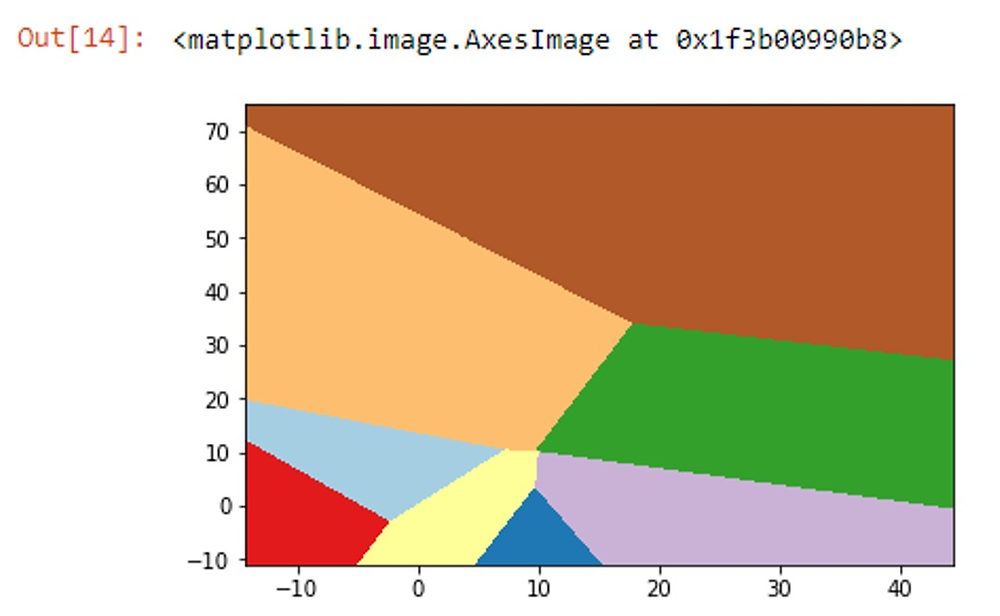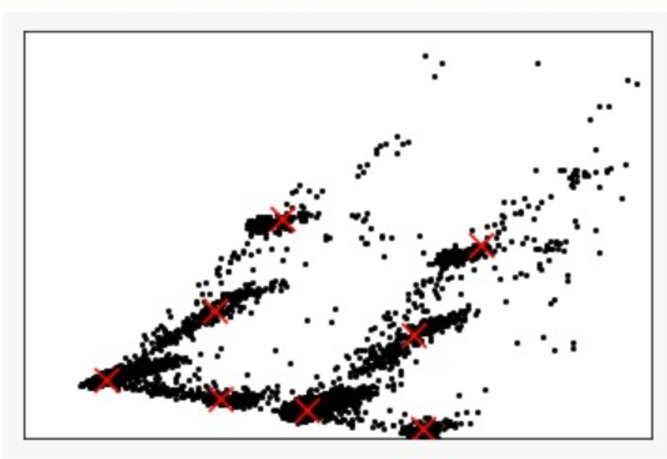Search

# Customer Segmentation via Machine Learning

Updated: Jul 28, 2020Depending on the amount of data you have and the number of variables you want to focus on in your analysis, cluster analysis may be the perfect tool for you. Especially when it comes to improving your user experience, cluster analysis can help you discover the pattern of people you are trying to market to by pulling in more data and creating an unbiased picture of your digital property. Simply put, it’s a way to discover insights from your data based on groups that are formed.

## An Introduction to Cluster Analysis

So how is cluster analysis different from segmenting? Think of segmenting as an umbrella term, with cluster analysis as one of many ways to segment your data. Cluster analysis is a tool that can segment your users based on behavior/tendencies, interests, age range, and more. The algorithm used is based on machine learning principles, but you have the option to define how many groups you want. Depending on the method, you can pick a number of desired clusters, or let the algorithm figure out how many distinct groups you have within your data.

# “Individual household electric power consumption”

## Lifecycle1. DEFINE PROBLEM AND DATA COLLECT- The problems definition steps and collecting data have already been performed.

2. PREPARE DATA - Normalization process and missing values treatment has been applied before. Anyway, as we find only 1,25% missing values, this does not generate much interference.

3. TRAIN MODEL – For all models, we apply the training procedure (70%) and test (30%)

4. EVALUATE - The k value minimum considered is 70%.

5. DEPLOY AND IMPROVE – This process considers future applications and adjustments, considering the entry of new future data.

Problem Description

The objective of this project is to identify patterns of consumption behavior based on historical data on electrical energy consumption by applying the machine learning method in order to support making decision in the formulation of public energy policies.

To solve this case, we will be using the most widely used unsupervised machine learning method called k-mean.

K-mean is a very intuitive solution and aims to group the dataset and apply a clustering technique, returning the similarities identified with the patterns within the attributes of the dataset, being extremely useful, because it allows the automatic search for patterns that are not perceived The value of k . We can define the k value considering the business rule and the personal evaluation based on dataset knowledge. The key point to the success of any predictive modeling project is in the pre-processive and treatment phase of dataset.

Data Set Information

This archive contains 2075259 measurements gathered in a house located in Sceaux (7km of Paris, France) between December 2006 and November 2010 (47 months).Attribute Information

1.date: Date in format dd/mm/yyyy 2.time: time in format hh:mm:ss 3.global_active_power: household global minute-averaged active power (in kilowatt) 4.global_reactive_power: household global minute-averaged reactive power (in kilowatt) 5.voltage: minute-averaged voltage (in volt) 6.global_intensity: household global minute-averaged current intensity (in ampere) 7.sub_metering_1: energy sub-metering No. 1 (in watt-hour of active energy). It corresponds to the kitchen, containing mainly a dishwasher, an oven and a microwave (hot plates are not electric but gas powered). 8.sub_metering_2: energy sub-metering No. 2 (in watt-hour of active energy). It corresponds to the laundry room, containing a washing-machine, a tumble-drier, a refrigerator and a light. 9.sub_metering_3: energy sub-metering No. 3 (in watt-hour of active energy). It corresponds to an electric water-heater and an air-conditioner.

The k-means application pre requisite is that the entire database to be used is in numerical form, for this purpose follows a small sample of this verification.

Otherwise, we must to convert the dataset for a numeric format before start to apply the k-means model.## Pre-processing Steps

The data does have missing values about 1.25% of the rows (about 82 days). We can see below some dates where we have missing values.

We can notice that the number of missing values is relatively small compared to the size of the database. So, it’s not harmful to the model if we delete these missing values.

```Python code :
power_consumption = data.iloc[0:, 2:9].dropna()```

Training and test the datasets Process

As we work with datasets, a machine learning algorithm works in two stages. We usually split the data around 30%-70% between testing and training stages.

```Python code :
pc_toarray = power_consumption.values
df_treino, df_teste= train_test_split(pc_toarray, train_size =.01)```

Dimensionality reduction

Applies dimensionality reduction, we are not reducing the numbers of variables

we only collected all variances from the variables and input it in 2 components

and these components represents the same variables information

so now we can work with only these 2 components to make predictions.

```Python code :
hpc = PCA(n_components = 2).fit_transform(df_train)```

Building the model

Now we apply the fit in the object calls hpc which was transformed before, with the reduced dimensionality.

```Python code :
k_means = KMeans()
k_means.fit(hpc)```

As we did not choose the parameters for k, the k_means() chose n_cluster=8 as a default, how we can see bellow.

Organizing the Data Cluster

Now we are going to organize the data to get minimum and maximum values and arrange shape to plot it and to build the graphic.

```Python code :
x_min, x_max = hpc[:, 0].min() - 5, hpc[:, 0].max() - 1
y_min, y_max = hpc[:, 1].min(), hpc[:, 1].max() + 5
xx, yy = np.meshgrid(np.arange(x_min, x_max, .02), np.arange(y_min, y_max, .02))
z = k_means.predict(np.c_[xx.ravel(), yy.ravel()])
z = z.reshape(xx.shape)```

Area Clusters Plot

This second process used k_means with k = 8.

Each color area represent a cluster area.

```Python code :
plt.figure(1)
plt.clf()
plt.imshow(z,
interpolation = 'nearest',
extent = (xx.min(), xx.max(), yy.min(), yy.max()),
cmap = plt.cm.Paired,
aspect = 'auto',
origin = 'lower')```Plot of each centroid’s clusters

The centroid or geometric center of a flat figure below is the arithmetic mean position of all points in the figure. The red X represent the 8 centroids clusters and we have some distant points that could not be identified. It is not a clustering mistake but show us that these points do not have similarities with the other groups, so it’s could be out liers or wrong data inserted to dataset.

So, in this case we should to discuss about it with the business area to be sure why these points are different.

```Python code :
plt.plot(hpc[:, 0], hpc[:, 1], 'k.' , markersize = 4)
centroids = k_means.cluster_centers_
inert = k_means.inertia_
plt.scatter(centroids[:, 0], centroids[:, 1], marker = 'x' , s = 169, linewidths = 3, color ='r', zorder = 8)
plt.xlim(x_min, x_max)
plt.ylim(y_min, y_max)
plt.xticks(())
plt.yticks(())

```Determining a range for K

In this specific case is possible to run this process, but in a big data case it would be hard to process it because it will spend to much time to run different k values.

```Python code :
k_range = range(1, 14)```

Appling K-Means model for each K value

The objective is to see the different results and identify the best k value using a list comprehension, running in one command different operations, creating a model and fitting it for each k value inside of the range.

```Python code :
k_means_var = [KMeans(n_clusters = k).fit(hpc) for k in k_range]```

Adjusting the centroids cluster for each model

The objective in this code using a list comprehension is collect the centroids for each model with different k values.

```Python code :
centroids = [X.cluster_centers_ for X in k_means_var]```

Calculating the Euclidean distance

For each centroids value listed before, we calculate the Euclidean distance (or metric distance ). The Euclidean distance is the distance between two points which can be proved by repeated application of the Pythagorean theorem.

```Python code :
k_euclid = [cdist(hpc, cent, 'euclidean') for cent in centroids]
dist = [np.min(ke,axis=1) for ke in k_euclid]```

Sum of the squares of distances inside of cluster

```Python code :
wcss = [sum(d**2) for d in dist]
Total Sum of the squares
tss = sum(pdist(hpc)**2)/hpc.shape```

Total Sum of the Squares - Sum of the Squares of distances inside of clusters

```Python code :
bss = tss - wcs```

Elbow Curv

The next step is calculate the variance resulted of the Total Sum of the Squares - Sum of the Squares of distances inside of clusters. From this result we know that for each k value we can understand the variance value

```Python code :
fig = plt.figure()
ax.plot(k_range, bss/tss*100, 'b*-')
ax.set_ylim((0,100))
plt.grid(True)
plt.xlabel('Numbers of clusters')
plt.ylabel('Variance Percent Explicated')
plt.title('Explicated Variance x K Value')```The Explicated Variance x K Value graphic show us that for k value >= 4 the variance could be more explicated

Creating a new clustering model with k = 7 .

```Python code :
k_means = KMeans(n_clusters = 7)
k_means.fit(hpc)```

Get the Minimum and Maximum Value and arrange the Shape.

```Python code :
x_min, x_max = hpc[:, 0].min() - 5, hpc[:, 0].max() - 1
y_min, y_max = hpc[:, 1].min(), hpc[:, 1].max() + 5
xx, yy = np.meshgrid(np.arange(x_min, x_max, .02), np.arange(y_min, y_max, .02))
z = k_means.predict(np.c_[xx.ravel(), yy.ravel()])
z = z.reshape(xx.shape)```

Area Clusters Plot.

```Python code :
plt.figure(1)
plt.clf()
plt.imshow(z,
interpolation = 'nearest',
extent = (xx.min(), xx.max(), yy.min(), yy.max()),
cmap = plt.cm.Paired,
aspect = 'auto',
origin = 'lower')

```Plot of each centroids clusters.

```Python code :
plt.plot(hpc[:, 0], hpc[:, 1], 'k.' , markersize = 4)
centroids = k_means.cluster_centers_
inert = k_means.inertia_
plt.scatter(centroids[:, 0], centroids[:, 1], marker = 'x' , s = 169, linewidths = 3, color ='r', zorder = 8)
plt.xlim(x_min, x_max)
plt.ylim(y_min, y_max)
plt.xticks(())
plt.yticks(())
plt.show()

```There was not much difference in outcome between the 7 and 8 cluster models.

Therefore, the cluster number is not always the most important in the clustering process,

but rather the interpretation collected from the model result.

Therefore, in-depth knowledge of the data and the business problem becomes important.

In this case, the clustering model application becomes the fastest part process

and the other parts of the process are those that require the most time

``` Python code :
?silhouette_score```

The best value is 1 and the worst value is -1. Values close to 0 indicate overlapping clusters, meaning that they belong to more than one cluster.Negative values usually indicate that a sample was assigned to the wrong cluster because a different cluster is more similar.

### Silhouette_score

```Python code :
labels = k_means.labels_
silhouette_score(hpc, labels, metric = 'euclidean')```## What are the benefits of customer segmentation?

#### 1. IT ALLOWS YOU TO FINE-TUNE YOUR MESSAGE

When you segment your marketing efforts to specific groups of people, it allows you to hone in on specific messages that you want to advertise.

It enables you to fine-tune your marketing message to align with exactly what the recipient is looking for, and therefore, increases the chances that they’ll convert.

By fine-tuning your marketing message, you’ll see increases in your revenue because users will be more likely to make a purchase when they’re delivered exactly what they need.

In fact, segmented and targeted emails generate 58% of all revenue for a company, which isn’t hard to believe.

When you segment your emails, you’ll also have a subject line that that is personalized to the recipients’ needs, which can increase open rate by 26%. And it’s obvious that the more emails are opened, the more sales you’ll make.

#### 3. YOU’LL INCREASE AWARENESS FOR YOUR BRAND

Users love personalized emails. When they receive something that’s made just for them, they’ll feel more comfortable purchasing from your company because they know you care.

This also builds brand awareness because customers will remember you as the company that sends them emails based on their interests, previous spending habits, and more.

39 views